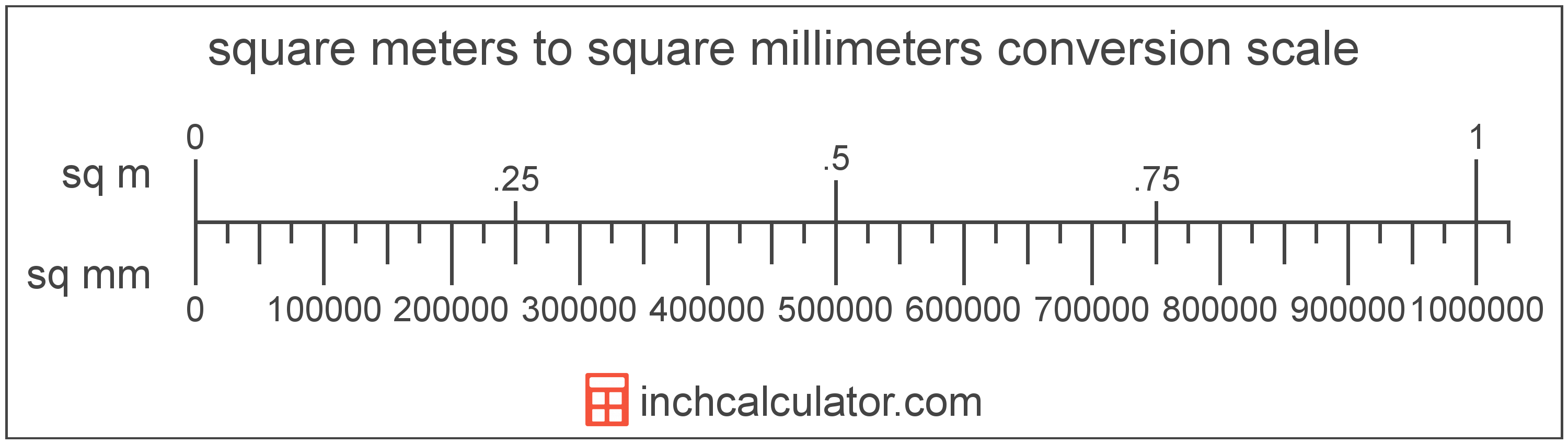# Square Meters to Square Millimeters Converter

Enter the area in square meters below to get the value converted to square millimeters.

(find square meters)
Results in Square Millimeters:1 sq m = 1,000,000 sq mm
1 sq m = 10,000 sq cm

Do you want to convert square millimeters to square meters?

## How to Convert Square Meters to Square Millimeters

To convert a measurement in square meters to a measurement in square millimeters, multiply the area by the following conversion ratio: 1,000,000 square millimeters/square meter.

Since one square meter is equal to 1,000,000 square millimeters, you can use this simple formula to convert:

square millimeters = square meters × 1,000,000

The area in square millimeters is equal to the area in square meters multiplied by 1,000,000.

For example, here's how to convert 5 square meters to square millimeters using the formula above.
square millimeters = (5 sq m × 1,000,000) = 5,000,000 sq mm### How Many Square Millimeters Are in a Square Meter?

There are 1,000,000 square millimeters in a square meter, which is why we use this value in the formula above.

1 sq m = 1,000,000 sq mm

## What is a Square Meter?

One square meter is equivalent to the area of a square with sides that are each 1 meter in length. One square meter is equal to 10.76391 square feet or 10,000 square centimeters.

The square meter, or square metre, is the SI derived unit for area in the metric system. A square meter is sometimes also referred to as a square m. Square meters can be abbreviated as sq m, and are also sometimes abbreviated as . For example, 1 square meter can be written as 1 sq m or 1 m².

You can use a square meters calculator to calculate the area of a space if you know its dimensions.

## What is a Square Millimeter?

One square millimeter is equivalent to the area of a square with edges that are each 1 millimeter in length. One square millimeter is equal to 0.00155 square inches or 1/100 of a square centimeter.

The square millimeter, or square millimetre, is a multiple of the square meter, which is the SI derived unit for area. In the metric system, "milli" is the prefix for thousandths, or 10-3. A square millimeter is sometimes also referred to as a square mm. Square millimeters can be abbreviated as sq mm, and are also sometimes abbreviated as mm². For example, 1 square millimeter can be written as 1 sq mm or 1 mm².

## Square Meter to Square Millimeter Conversion Table

Table showing various square meter measurements converted to square millimeters.
Square Meters Square Millimeters
0.000001 sq m 1 sq mm
0.000002 sq m 2 sq mm
0.000003 sq m 3 sq mm
0.000004 sq m 4 sq mm
0.000005 sq m 5 sq mm
0.000006 sq m 6 sq mm
0.000007 sq m 7 sq mm
0.000008 sq m 8 sq mm
0.000009 sq m 9 sq mm
0.0000001 sq m 0.1 sq mm
0.000001 sq m 1 sq mm
0.00001 sq m 10 sq mm
0.0001 sq m 100 sq mm
0.001 sq m 1,000 sq mm
0.01 sq m 10,000 sq mm
0.1 sq m 100,000 sq mm
1 sq m 1,000,000 sq mm

## References

1. Collins Dictionary, Definition of 'square meter', https://www.collinsdictionary.com/us/dictionary/english/square-meter